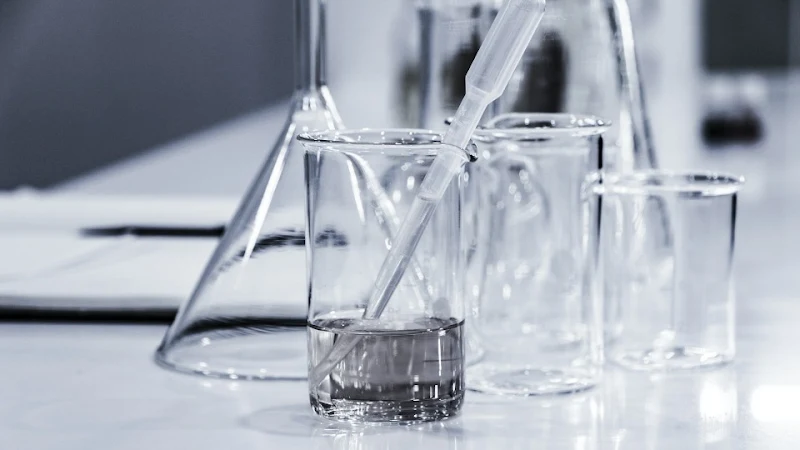# 【DSE CHEM】Chem DSE Mole Calculation 莫爾數攻略 | 附常見問法Mole Calculation 雖然係一個中四 Level 嘅 DSE Chem Topic，但其實有好多同學仔見到 Mole Calculation 嘅題型，都真係無從入手。其實原因好簡單：因為你無一個有系統嘅「Mole 數」思維，去幫助你應對 DSE Chem 題目。

## 咩係「莫爾數 Mole Calculation」?

Photo by Terry Vlisidis on Unsplash

Mole Calculation 喺 DSE Chem syllabus 裡面，其實涵蓋咗好多唔同嘅 Sub-topics，例如：
• 3. Metals：Molar Mass, Empirical Formula
• 4. Acids & Bases：Molarity, Dilution, Volumetric Analysis
• 8. Energy：Enthalpy Change
• 9. Rate of Reaction：Molar Volume⋯⋯

## 有邊啲必記嘅 Mole Calculation Formula？

Photo by Bill Oxford on Unsplash

## Mole Calculation 常見於邊啲 DSE Chem Topics？

Photo by Lesley Davidson on Unsplash

### Metals（金屬）

Metal 呢個 DSE Chem Topic 會出好多 Metal Reaction equation 同 reaction 嘅題目，甚至出返好耐之前學嘅 Empirical Formula 考你點樣計。

## 點先可以做好莫爾數計算 (Mole Calculation)？

### 搵出唔同嘅 Variables 扣連公式

Photo by Roman Mager on Unsplash

### 改變閱讀題目嘅順序

Photo by Joel Muniz on Unsplash

### 將成個實驗過程畫出嚟

Photo by Julia Koblitz on Unsplash

✅ 6 大 DSE 必出 MOLE 數題型嘅拆題思維
✅ 深入分析考評出卷 Pattern
✅ 最強高效 DSE CHEM 奪星溫習法

## 必做嘅幾條 Mole Calculation Exercise （附詳細解題）

### 題型一：Percentage by Mass（質量百分比）

Calculate the percentage by mass of copper in copper(II) oxide (CuO).
(Relative atomic masses: Cu = 62.9; O = 16.0)

Step 1: 搵出主角

Step 2: 將相關數值放入公式

### 題型二：Mole Ratio（摩爾比）

Consider the following reaction:
Calculate the mass of lead oxide required to produce 89 g of lead.
(Relative atomic masses: O = 16.0, Pb = 207.0)

Step 1: 先拆題

Mass of Pb₃O₄ ← Mole of Pb₃O₄ ← Mole of Pb ← Mass of Pb

Step 2: 計算 Mole of Pb

Step 3: 計算 Mole of Pb₃O₄

Pb : Pb₃O₄ = 3 : 1

Step 4: 計算 Mass of Pb₃O₄

### 題型三：Limiting Reagent（有限反應物）

When 16.28 g of zinc oxide are heated with 5.23 g of carbon powder, zinc will be formed. Write down a balanced equation and calculate the mass of zinc formed.
(Relative atomic masses: C = 12.0; O = 16.0; Zn = 65.4)

Step 1: 先寫出 Balanced Equation

Step 2: 拆題並搵出 Limiting Reagent

Step 3: 利用 Limiting Reagent 去完成接下來嘅計算

### 題型四：Empirical Formula（實驗式）

After conducting an experiment on an unknown compound X, it was found that X only 70% iron and 30% oxygen by mass. What is the empirical formula of X.
(Relative atomic masses: Fe = 55.8; O = 16.0)

Step 1: 搵出主角

Step 2: 喺 Table 上寫出主角，再搵埋佢地嘅 Mass
Table 主要會分為三行：Mass, Mole 和 Mole Ratio，你一定要先分別搵到主角們嘅 mass， 先可以做下一步！但正常情況下，題目唔會直接將 mass 列出嚟，但會提供每個 element 嘅 % by mass！所以你可以先 “Let the mass of compound X be 100 g.”，咁樣你就可以輕鬆搵到 Fe 和 O 嘅 mass 喇！

Step 3: 分別搵出主角嘅 Mole 數

Step 4: 搵出主角間嘅 Mole Ratio

Step 5: 約簡 Mole Ratio 再搵出 Empirical Formula

Fe : O = 2 : 3 ➝ Empirical Formula is Fe₂O₃.The Chemist🧪

🚀定期分享 DSE CHEM 嘅學習心得、應試技巧🧪港大化學系畢業．專科專教🎖港大理碩士學歷．鑽研最強教學法🏅曾與港大教授進行兩年教育研究🏆多年DSE教學經驗．曾教授過千學生